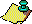Contents Next: Sources Up: RamanujanModular Equations, Previous: Modular Equations and

# Ramanujan's sum

This amazing sum,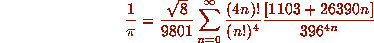is a specialization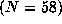of the following result, which gives reciprocal series forin terms of our function alpha and related modular quantities.
[More Ramanujan's type series are recorded with in ...]

### Theorem 5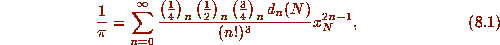where,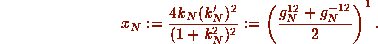with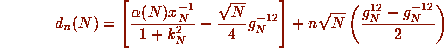and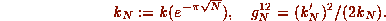Here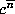is the rising factorial: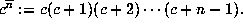Some of the ingredients for the proof of Theorem 5, which are detailed in , are the following. Our first step is to write (7.2) as a sum after replacing the E by K and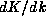using (5.7). One then uses an identity of Clausen's which allows one to write the square of a hypergeometric function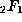in terms of a generalized hypergeometric, namely, for all k one has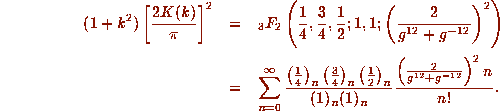Here g is related to k by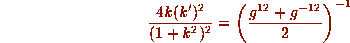as required in Theorem 5. We have actually done more than just use Clausen's identity, we have also transformed it once using a standard hypergeometric substitution due to Kummer. Incidentally, Clausen was a nineteenth-century mathematician who, among other things, computed 250 digits ofin 1847 using Machin's formula. The desired formula (8.1) is obtained on combining these pieces.

Even with Theorem 5, our work is not complete. We still have to compute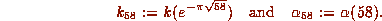In fact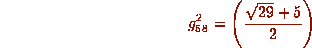is a well known invariant related to the fundamental solution to Pell's equation for 29 and it turns out that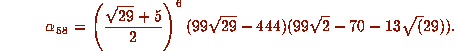One can, in principle, and for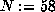, probably in practice, solve for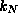by directly solving the Nth-order equation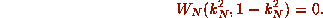For N=58 given that Ramanujan  and Weber  have calculated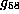for us, verification by this method is somewhat easier though it still requires a tractable form of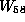. Actually, more sophisticated number-theoretic techniques exist for computing(these numbers are called singular moduli). A description of such techniques, including a reconstruction of how Ramanujan might have computed the various singular moduli he presents in  is presented by Watson in a long series of papers commencing with ; and some more recent derivations are given in  and . An inspection of Theorem 5 shows that all constants in Series 1 are determined from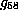. Knowingis equivalent to determining that the number 1103 is correct.

It is less clear how one explicitly calculates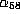in algebraic form, except by brute force, and a considerable amount of brute force is required; but a numerical calculation to any reasonable accuracy is easily obtained from (7.3) and 1103 appears! The reader is encouraged to try this to, say, 16 digits. This presumably is what Ramanujan observed. Ironically, when Gosper computed 17 million digits ofusing Sum 1, he had no mathematical proof that Sum 1 actually converged to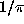. He compared ten million digits of the calculation to a previous calculation of Kanada et al. This verification that Sum 1 is correct to ten million places also provided the first complete proof that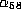is as advertised above. A nice touch --- that the calculation of the sum should prove itself as it goes.

Roughly this works as follows. One knows enough about the exact algebraic nature of the components of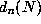and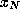to know that if the purported sum (of positive terms) were incorrect, then before one reached 3 million digits, this sum must have ceased to agree with. Notice that the components of Sum 1 are related to the solution of an equation of degree 58, but virtually no irrationality remains in the final packaging. Once again, there are very good number-theoretic reasons, presumably unknown to Ramanujan, why this must be so (58 is at least a good candidate number for such a reduction). Ramanujan's insight into this marvelous simplification remains obscure. Ramanujan  gives 14 other series for, some others almost as spectacular as Sum 1 --- and one can indeed derive some even more spectacular related series.( Added in proof) Many related series due to Borwein and Borwein and to Chudnovsky and Chudnovsky appear in papers in Ramanujan Revisited, Academic Press, 1988.

He almost gives no explanation as to their genesis, saying only that there are ``corresponding theories'' so the standard theory (as sketched in section 5) from which they follow. Hardy, quoting Mordell, observed that ``it is unfortunate that Ramanujan has not developed the corresponding theories''. By methods analogous to those used above, all his series can be derived from the classical theory . Again it is unclear what passage Ramanujan took to them, but it must in some part have diverged from ours.

We conclude by writing down another extraordinary series of Ramanujan's, which also derives from the same general body of theory.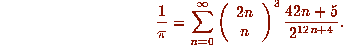This series is composed of fractions whose numerators grow like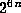and whose denominators are exactly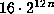. In particular this can be used to calculate the second block of n binary digits ofwithout calculating the first n binary digits. This beautiful observation, due to Holloway, results, disappointingly, in no intrinsic reduction in complexity.Contents Next: Sources Up: RamanujanModular Equations, Previous: Modular Equations and